Courses

# RD Sharma Solutions - Chapter 1 - Rational Numbers (Ex - 1.3), Class 8, Maths Class 8 Notes | EduRev

## RD Sharma Solutions for Class 8 Mathematics

Created by: Abhishek Kapoor

## Class 8 : RD Sharma Solutions - Chapter 1 - Rational Numbers (Ex - 1.3), Class 8, Maths Class 8 Notes | EduRev

The document RD Sharma Solutions - Chapter 1 - Rational Numbers (Ex - 1.3), Class 8, Maths Class 8 Notes | EduRev is a part of the Class 8 Course RD Sharma Solutions for Class 8 Mathematics.
All you need of Class 8 at this link: Class 8

Question 1: Subtract the first rational number from the second in each of the following: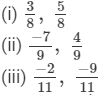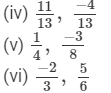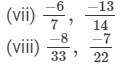(i)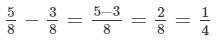(ii)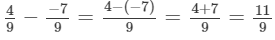(iii)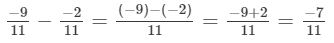(iv)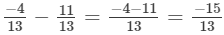(v)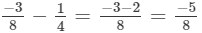(vi)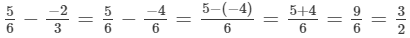(vii)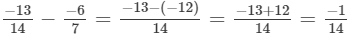(viii)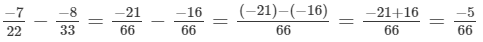Question 2: Evaluate each of the following: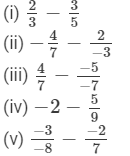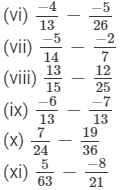(i)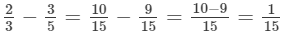(ii)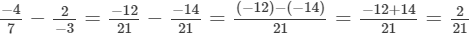(iii)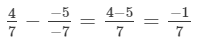(iv)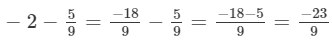(v)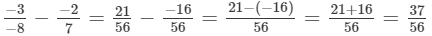(vi)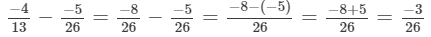(vii)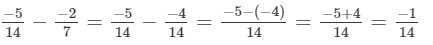(viii)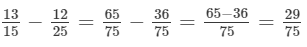(ix)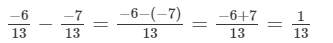(x)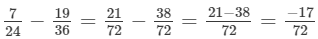(xi)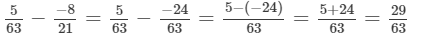Question 3: The sum of the two numbers is 5/9.59. If one of the numbers is 1/3,13, find the other.

It is given that the sum of two numbers is 5/9, where one of the numbers is 13.Let the other number be x.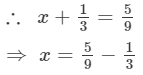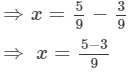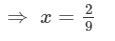Question 4: The sum of two numbers is -1/3.-13. If one of the numbers is -12/3,-123, find the other.

Answer : It is given that the sum of two numbers is-1/3, where one of the numbers is −12/3.Let the other number be x.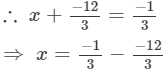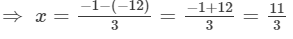Question 5: The sum of two numbers is -4/3.-43. If one of the numbers is −5, find the other.

It is given that the sum of two numbers is −4/3, where one of the numbers is −5.Let the other number be x.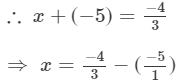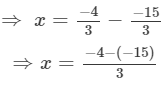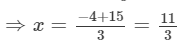Question 6: The sum of two rational numbers is −8. If one of the numbers is -15/7,-157, find the other.

It is given that the sum of two rational numbers is −8, where one of the numbers is −15/7.Let the other rational number be x.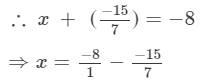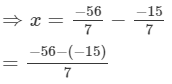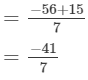Therefore,theotherrationalnumberis -41/7.

Question 7: What should be added to -7/8 so as to get 5/9?

Answer : Let x be added to -7/8 so as to get 5/9.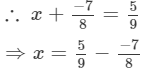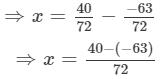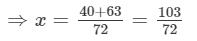Question 8: What number should be added to -5/11-511 so as to get 26/33 ?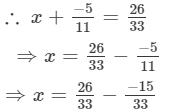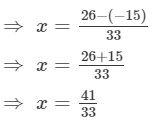Question 9: What number should be added to -5/7 to get -2/3?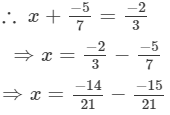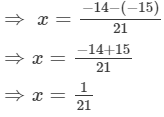Question 10: What number should be subtracted from -5/3  to get 5/6?
Answer : Let x be subtracted.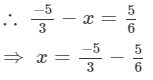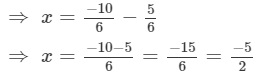Question 11:What number should be subtracted from 3/7 to get 5/4?

Answer : Let, x be subtracted.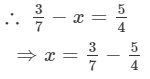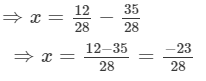Question 12: What should be added to (2/3+3/5) to get −2/15?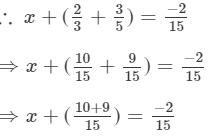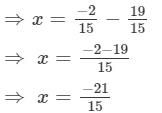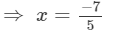Question 13: What should be added to (1/2+1/3+1/5) to get 3?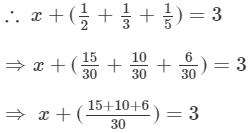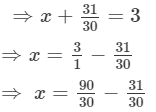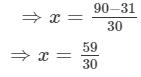Question 14:What should be subtracted from (3/4−2/3) to get −1/6?
Let x be subtracted.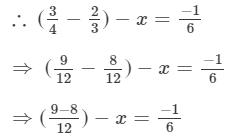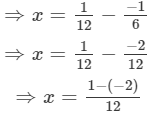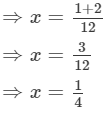Question 15: Fill in the blanks: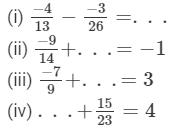(i)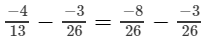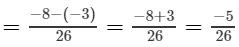(ii)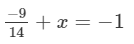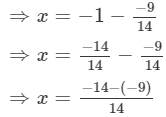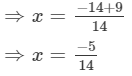(iii)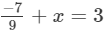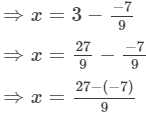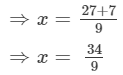(iv)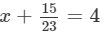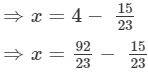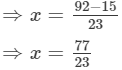91 docs

,

,

,

,

,

,

,

,

,

,

,

,

,

,

,

,

,

,

,

,

,

,

,

,

,

,

,

;